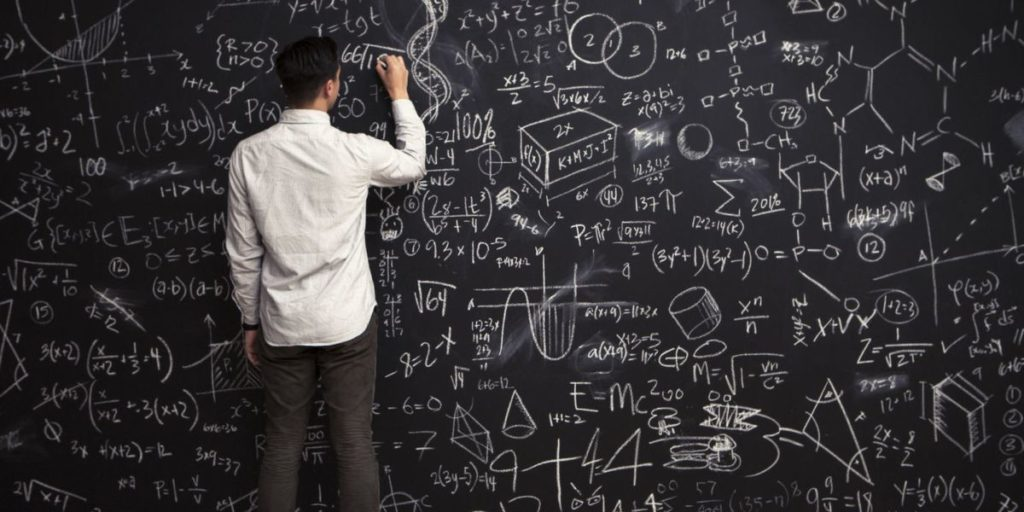Archives for

# Most Confusing Math

## What Are Some of The Most Confusing Math Problems Ever?

A problem which is presented, analyzed and solved with the help of the mathematical methods is known as a mathematical problem. The nature of this problem can be real or abstract. The best example of the real world mathematical problem is to construct the orbit of the planets in the solar system. On the other hand, Russell’s Paradox is the best example of the abstract mathematical problem. After solving the mathematical problem, we are able to express its result formally. There are some mathematical problems which are confusing to us. Some examples of the most confusing math problems are given below;• Problem with fruit

There are some people who provide some specific values to different fruits and try to ask some confusing questions on the basis of these values. For example, if a person says that if the value of three bananas is thirty, the value of four apples is 60 and the value of two coconuts is equal to 40, what will be the value of the following equation;

Apple + Banana + Coconut = ?

The value of this equation will be equal to 45.

In a similar way, people also make such problems which are confusing for people. Most of the people are not able to solve these confusing problems.

• Missing dollar

This equation is explained in various ways. Now, we try to explain it in the simplest way. For example, I want to buy a book and its price is \$97. My mother gave me \$50 and my father had also given \$50 to me. I have paid \$97 dollars to the bookseller and I have returned one dollar to my mother and one dollar to my father. Moreover, I have also kept one dollar. Now, I have owed \$49 from my mother and \$49 dollars from my father. Now, I calculate these dollars in the following way;

\$49 + \$49 + \$1 = \$99.

Now, the confusion is that I had \$100 and one dollar is missing from my calculation. Most of the people are not able to solve this simple equation.

• People on the train

There are also some questions that are asked in different standards mathematical papers and it is hard for the students to find out the solutions to these questions. For example, a confusing question was asked in the second degree equivalent test. According to this question, there were some people on the train. At the first station, 21 people get out of the train and 23 people get on the train at the same station. Now, there are 75 people in the train. Find out the total number of people on the train when it began? The answer to this question is very simple. The answer to this question is that there were 73 people on the train when it began.

In a similar way, the people also face some problems to solve some other confusing math problems like happy birthday questions, more Pemdas, parking spot number, broken water heater and three line equations etc.